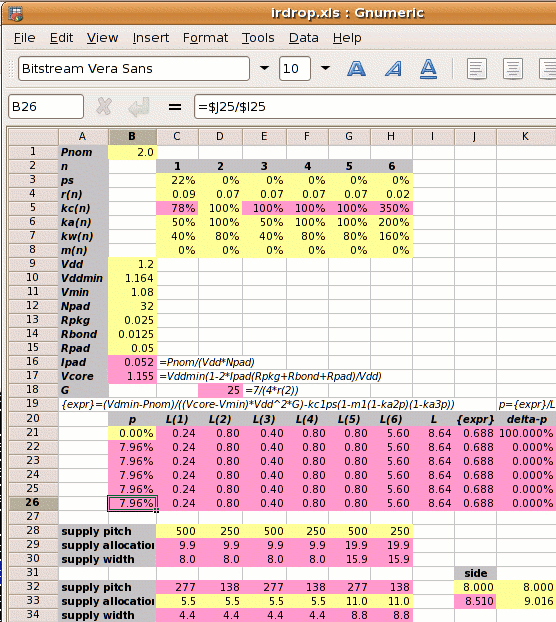Step 1: Calculate Ipad and Vcore:

1⁄(1.2×16) = 0.052A
Vcore =
1.164×(1−2×0.052×(0.025+0.0125+0.05)⁄1.2
1.155V

Step 2: Calculate the reference power supply conductance G:

G =
 7 4×r2

7 ⁄ (4 × 0.07) =  25 mhos

Step 3 is to set out the values of kan, kwn, kcn and mn for each metal layer, and use these to calculate the value of L.

metal layer 1 2 3 4 5 6
kan  50% 100%  50% 100%  50% 200%
power metal allocated coefficient
kwn  80%  80%  80%  80%  80%  80%
power metal used coefficient
kcn  78%¹ 100% 100% 100% 100% 350%²
conductivity coefficient
mn³   0%   0%   0%   0%   0%   0%
core area blocked
¹78%=.07/.09; ²350%=.07/.02; ³no fixed blocks

Normally we can't calculate L directly because its value depends on p which we don't know. But in this case with m1‑6=0 we can

 L = kw1kc1(1-ps)(1-m1(1-ka2p)(1-ka3p))+ kw2kc2(1-m2(1-ka2p)(1-ka3p))+ kw3kc3(1-m3(1-ka2p)(1-ka3p))+ kw4kc4(1-m4(1-ka2p)(1-ka3p))+ kw5kc5(1-m5(1-ka2p)(1-ka3p))+ kw6kc6(1-m6(1-ka2p)(1-ka3p)) = ( 0.24 + 0.8 + 0.4 + 0.8 + 0.8 + 5.6 ) = 8.64

Step 4: Calculate the power strap allocation percentage p:

p =
 { Vddmin×Pnom −kc1×ps } × 1 (Vcore−Vmin)×Vdd2×G L
 { 1.164×2 −0.78×0.22 } × 1 (1.155−1.08)×1.22×25 8.64
(0.860−0.173)×0.116 = 7.96%

As shown on the right, a spreadsheet can be used to iterate to the answer.

Step 5: Calculate the new core size. If the initial core size estimate without power straps is x, then with power straps the core size becomes x

 x′ = x = x = x+6.37% √(((1−ka2p)(1−ka3p)) √(0.9204×0.9602)

The value 6.37% is called the IR Drop Adder.

The width of the power straps is set by the pitch strap allocation or width chosen by the user and the value of p just calculated. An example is shown in the table below, where we set the supply strap allocation to 5.5µm and compare it to the old solution.

p allocation metal pitch core
1-4 5 6 hor ver side
old 14.92% 5.5µm 5.5µm 11µm 147µm  74µm 9.016mm
new  7.96% 5.5µm 11µm 11µm 277µm 138µm 8.510mm

Design Attribute Value
Pnom core power consumption 2W
ps fraction of metal-1 in the standard cells used for power supplies 22% (for vsclib)
r1 resistivity of metal layer 1 in ohms per square 0.09Ω per sq.
r2-5 resistivity of metal layers 2-5 in ohms per square 0.07Ω per sq.
r6 resistivity of metal layer 6 in ohms per square 0.02Ω per sq.
ka1,3,5
 user defined   ratio of metal layers 1,3,5 allocated to power metal-2 allocated to power
50%
ka4
 user defined   ratio of metal layer 4 allocated to power metal-2 allocated to power
100%
ka6
 user defined   ratio of metal layer 6 allocated to power metal-2 allocated to power
200%
mn percentage of metal layer n blocked to power straps 0%
Vdd the nominal supply voltage 1.2V
Vddmin the minimum supply voltage, 3% less than nominal 1.164V
Vmin the desired voltage at the centre of the die, 10% less than the nominal 1.08V
Rpkg the resistance of the package leadframe 25mΩ
Rbond the resistance of the bond wire 12.5mΩ¹
¹double bond halves bond wire resistance; ²two supply pads halves pad resistance

kcn =
 r2 rnThe new solution has allowed the vertical power strap pitch to go up from one every 74µm to one every 138µm. The core side is 506µm less and the core area is 11% less than the first solution, due to double bonding of supply pads, tighter Vddmin spec and wider metal-5 straps.